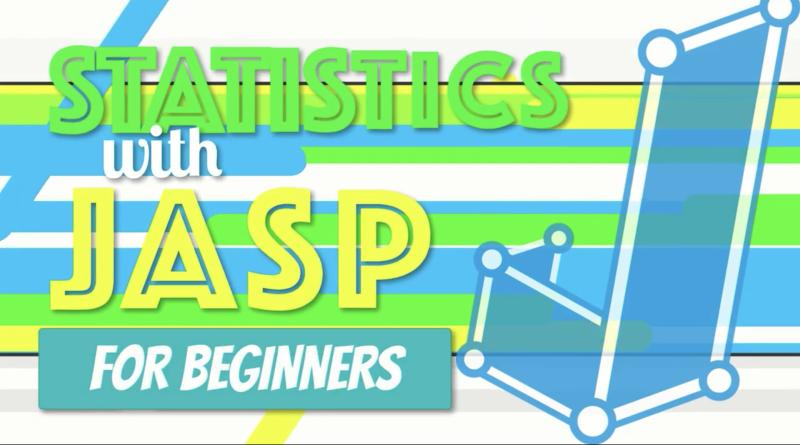# Video: How to Do Chi-Square in JASP

Video (10:27) from Research by Design in which, “We learn how to calculate a One-Way Chi-Square goodness of fit test in JASP using the setting for Multinomial Test. For the null hypothesis, we assume that the observed values in one group (the Pigs) do not differ from the values in a comparison population (the Bears) or that there is no difference between the group in their choice of building materials: Straw, Sticks, or Brick and mortar. We create a simple data set in Excel, then open the .CSV dataset in JASP. We conduct the test, interpret the results, and write up the findings in APA style. This is the Pig and Bear Chi Square.”

In this video (9:44) “We learn how to calculate a One-Way Chi-Square in JASP using the setting for Multinomial Test. For the null hypothesis, we assume that the observed values do not differ from randomness or that there is no preference among the three types of materials: Straw, Sticks, or Brick and mortar. We create a simple data set in Excel, then open the .CSV dataset in JASP. We conduct the test, interpret the results, and write up the findings in APA style. This is the House of Pigs Chi Square.”

If you’d like to have any of the files used in the video, you can access them here.

You can find other videos from the Statistics with JASP for Beginners series here.